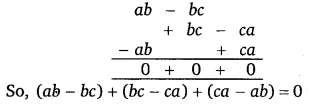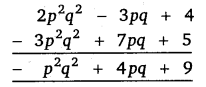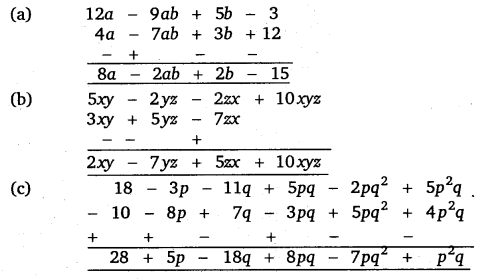# Class 8 Maths NCERT Solutions for Chapter – 9 Algebraic Expressions and Identities Ex – 9.1

### Algebraic Expressions and Identities

Question 1.
Identify the terms, their coefficients for each of the following expressions :
(i)
5xyz2 – 3zy
(ii) 1 + x + x2
(iii) 4x2y2 – 4x2y2z2 + z2
(iv) 3 – pq + qr -rp
(v)$\frac { x }{ 2 } +\frac { y }{ 2 } -xy$
(vi) 0.3a – 0.6ab + 0.5b

Solution:
(i) In the expression 5xyz2 – 3zy, the terms are 5xyz2 and -3zy.
Coefficient of xyz2 in the term 5xyz2 is 5.
Coefficient of zy in the term – 3yz is – 3.

(ii) In the expression 1 + x + x2, the terms are 1, x and x2.
Coefficient of term 1 is 1.
Coefficient of x in the term x is 1.
Coefficient of x2 in the term x2 is 1.

(iii) In the expression 4x y – 4xyz + z , the terms are 4x2y2, – 4x2y2z2 and z2.
Coefficient of x2y2 in the term 4x2y2 is 4.
Coefficient of x2y2z2 in the term – 4x2y2z2 is – 4.
Coefficient of z2 in the term z2 is 1.

(iv) In the expression 3 – pq + qr – rp, the terms are 3, – pq, qr and – rp.
Coefficient of term 3 is 3.
Coefficient of pq in the term – pq is -1.
Coefficient of qr in the term qr is 1.
Coefficient of rp in the term – rp is -1.

(v) In the expression$\frac { x }{ 2 } +\frac { y }{ 2 } -xy$, the terms are$\frac { x }{ 2 } ,\frac { 7 }{ 2 }$ and – xy.
Coefficient of x in the term$\frac { x }{ 2 }$ is$\frac { 1 }{ 2 }$
Coefficient of y in the term$\frac { y }{ 2 }$ is$\frac { 1 }{ 2 }$
Coefficient of xy in the term – xy is -1.

(vi) In the expression 0.3a -0.6 ab + 0.5 b, the terms are 0.3a, – 006ab and 0.5b.
Coefficient of a in the term 0.3a is 0.3.
Coefficient of ab in the term – 0.6ab is – 0.6.
Coefficient of b in the term 0.5b is 0.5.

Question 2.
Classify the following polynomials as monomials, binomials, trinomials. Which polynomials do not fit in any of these three categories?
x + y, 1000, x + x2 + x3 + x4, 7 + y + 5x, 2y – 3y2, 2y – 3y2 + 4y3, 5x – 4y + 3xy, 4z – 15z2, ab + bc+cd + da, pqr, p2q + pq2, 2p + 2q.

Solution:
The given polynomials are classified as under :
Monomials : 1000, pqr
Binomials : x + y, 2y – 3y2, 4z -15z2, p2q + pq2, 2p + 2q.
Trinomials : 7 + y + 5x, 2y – 3y2 + 4y3, 5x – 4y + 3x.
Polynomials that do not fit in any categories :
x + x2 + x3 + x4, ab + be + cd + da.

Question 3.
(i)
ab – be, be – ca, ea – ab
(ii) a – b + ab, b-c + be, c – a + ac
(iii) 2p2q2 – 3pq + 4, 5 + 7pq – 3p2q2
(iv) l2 + m2, m2 + n2, n2 + l2, 2Im + 2mn + 2nl

Solution:
(i) Writing the given expressions in separate rows with like terms one below the other, we have(ii) Writing the given expressions in separate rows with like terms one below the other, we have(iii) Writing the given expressions in separate rows with like terms one below the other, we have(iv) Writing the given expressions in separate rows with like terms one below the other, we haveQuestion 4.
(a) Subtract 4a – lab +36 + 12 from 12a – 9a6 + 56-3

(b) Subtract 3xy + 5yz – Izx from 5xy – 2yz – 2zx + IQxyz

(c) Subtract 4p2q – 3pq + 5pq2 – 8p + 7q – 10 from 18 – 3p – 11q + 5pq – 2pq2 + 5p2q.

Solution:
Rearranging the terms of the given expressions, changing the sign of each term of the expression to be subtracted and adding the two expressions, we get